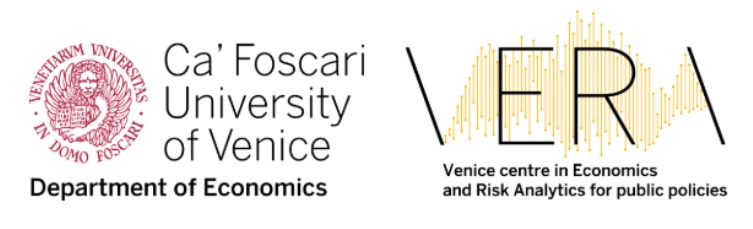The Italian Econometric Association (SIdE-IEA) in collaboration with the Venice centre in Economic and Risk Analytics for Public Policies (VERA) Ca’ Foscari University of Venice organizes the course for PhD students in:

Bayesian Methods in Economics and Finance

28 August -  1 September , 2023

Università Ca' Foscari  Venezia

Coordinator

Gaetano Carmeci
Università di Trieste
Dipartimento di Scienze Economiche, Aziendali, Matematiche e Statistiche “B. de Finetti” (DEAMS)
Via Tigor 22
34124 Trieste
tel.+39 0405587100
e-mail: gaetano.carmeci@deams.units.it

Lecturers

Gaetano Carmeci, University of Trieste
Roberto Casarin, University of Venice, Italy Ca' Foscari
Matteo Ciccarelli, European Central Bank, DG Economics, Head of Forecasting and Policy Modelling Division
Federico Bassetti, Politecnico di Milano

Basic Requirements

Intermediate knowledge of econometrics

The maximum number of allowed participants in presence is 20.

Description:

The course is an introduction on Bayesian Inference, starting from first principles and covering topics of interest for applied econometricians in economics and finance. The course is addressed to students without previous knowledge of Bayesian Econometrics. The methods introduced in the lectures will be illustrated with hands-on applications in MATLAB based on reasoned statistical and economic examples.

A. Fundamentals of Bayesian Statistics

B. Bayesian computation

•  Monte Carlo simulation

•  Markov chains

•  Markov Chain Monte Carlo methods (Gibbs sampler and Metropolis-Hastings algorithm)

a.  Comparing performance

b.  Checking convergence

c.   Optimal scaling

d.  An introduction to advanced MCMC and other simulation methods

C. Bayesian methods for regression models

a.  Standard LRM with spherical and non-spherical errors

b.  Hierarchical models

c.   Model checking and model choice

d.  Seemingly Unrelated Regression models

e.  Panel data models

f.    Introduction to time-varying parameter and stochastic volatility models

g.  Bayesian VAR models

D. Bayesian Nonparametric Methods

a.  Nonparametric Inference Methods

b.  Bayesian nonparametric data clustering

c.   Bayesian Nonparametric Lasso VAR

• Software

Participants will use their laptops with MATLAB already installed on them.

Reference textbook for the course:

• Berger, J. O. (1985), Statistical Decision Theory and Bayesian Analysis. Springer Series in Statistics (Second ed.). Springer Verlag.
• Gilks, W. R., S. Richardson and D. J. Spiegelhalter (1996), Markov chain Monte Carlo in practice, London: Chapman and Hall.
• Greenberg, E. (2008), Introduction to Bayesian Econometrics, Cambridge University Press.
• Koop, G. (2003), Bayesian Econometrics, J. Wiley.
• Koop, G., Dale J. P., Tobias, J. L. (2007.), Bayesian Econometric Methods, Cambridge University Press.
• Kroese, D.P. and J. Chan (2014), Statistical Modeling and Computation, Springer Verlag.
• Liu, J. (2001), Monte Carlo Strategies in Scientific Computing, Springer Verlag.
• Robert, C. P. (2001), The Bayesian Choice – A Decision-Theoretic Motivation (second ed.). Springer- Verlag.
• Robert, C.P. and Casella G. (2004), Monte Carlo Statistical Methods, New York: Springer Verlag.
• Robert, C.P. and Casella G. (2009), Introducing Monte Carlo Methods with R, New York: Springer Verlag.
• Zellner, A. (1971), Introduction to Bayesian Inference in Econometrics, Wiley and Sons.

More references in the public attachment section.

Venue and timetables

Each Module requires a full-time attendance and participation is not compatible with other jobs at the same time (e.g. preparation of other exams). Lectures and tutorials will be in English

• Monday to Friday: lectures: 9.00/9.30-13.00, 15.00-18.30.

The course will be held in the Campus Economico San Giobbe at Università Ca’ Foscari, Venezia, Italy. Address: Dipartimento di Scienze Economiche - S. Giobbe, 873 - 30121 Venezia.
Participants will be hosted in the Ca' Foscari Residence, inside the Campus Economico San Giobbe (in case of reduced availability of rooms they will be accommodated in local hotels).

Contacts